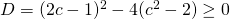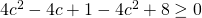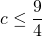# Solution assignment 05 Root equations

### Assignment 5

Given the line:and the function: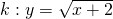For which values(s) of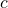doand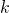have at least one point in common.

### Solution

In order to find possible intersection points we solve the following equation: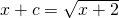We do not first look at the conditions for which the equation is defined but now try to solve the equation first and check whether the possible solutions satisfy the equation afterwards.
We square both sides of the equation: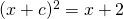andhave at least one point in common if the discriminant of this quadratic equation is greater than or equal to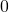, so: